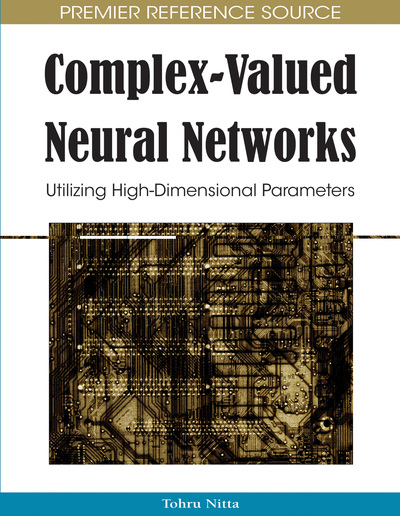# Image Reconstruction by the Complex-Valued Neural Networks: Design by Using Generalized Projection Rule

Donq-Liang Lee
DOI: 10.4018/978-1-60566-214-5.ch010
OnDemand:
(Individual Chapters)
Available
\$37.50
No Current Special Offers

## Abstract

New design methods for the complex-valued multistate Hopfield associative memories (CVHAMs) are presented. The author of this chapter shows that the well-known projection rule can be generalized to complex domain such that the weight matrix of the CVHAM can be designed by using the generalized inverse technique. The stability of the presented CVHAM is analyzed by using energy function approach which shows that in synchronous update mode a CVHAM is guaranteed to converge to a fixed point from any given initial state. Moreover, the projection geometry of the generalized projection rule is discussed. In order to enhance the recall capability, a strategy of eliminating the spurious memories is reported. Next, a generalized intraconnected bidirectional associative memory (GIBAM) is introduced. A GIBAM is a complex generalization of the intraconnected BAM (IBAM). Lee shows that the design of the GIBAM can also be accomplished by using the generalized inverse technique. Finally, the validity and the performance of the introduced methods are investigated by computer simulation.
Chapter Preview
Top

## Introduction

Storing gray-scale images with neural networks is a challenging problem and has received much attention in the past two decades. There are four main approaches for storing images with n pixels and K gray levels. The first approach is to encode the gray level of each pixel by R () binary neurons (Taketa & Goodman, 1986; Cernuschi-Frias, 1989; Lee, 1999). However, this method needs great numbers of neurons (nR) and interconnection weights (). The second approach is based on neural networks with multivalued stable states (Si & Michel, 1991; Zurada, Cloete, & van der Poel, 1996). The activation function is a quantized nonlinearity with K plateaus corresponding to the K gray levels. The required number of neurons is n and the number of interconnections is. The third approach is to decompose the K gray-level image into R gray-coded images (Costantini, Casali, & Pefetti, 2003). These images are then stored by using R independent binary neural networks. The required number of interconnections is. The fourth approach is based on complex-valued neural networks (Jankowski, Lozowski, & Zurada, 1996; Lee, 2001a, 2001b, 2003). The neuron state can assume one of K complex values, equally spaced on the unit circle. Each phase angle corresponds to a gray level. The number of neurons is n; the number of interconnections is.

The objective of this chapter is to review and discuss some recent developments of the complex-valued neural networks (CVNNs). Here we present two types of CVNNs: auto-associative network and hetero-associative network. Network structures, evolution equations, stability, and design methods are discussed in detail. For simplicity, the CVNNs considered here have no threshold (bias) vectors though the including of them adds substantial degree of freedom to the design

The complex-valued Hopfield associative memory (CVHAM) proposed by Jankowski, Lozowski, and Zurada (1996) is a kind of auto-associative network. It can be referred to as a modified Hopfield network (Hopfield, 1984) having complex-signum activation functions and complex weighting connections. The learning algorithms of conventional CVHAMs include: generalized Hebb rule (Jankowski, Lozowski, & Zurada, 1996), gradient descent learning rule (Lee, 2001a, 2003), energy design method (Müezzinoğlu, Güzeliş, & Zurada, 2003), etc. However, the recall capability of the CVHAMs is limited because these methods do not consider the attraction of the fixed point seriously. In this chapter, the generalized projection rule for the CVHAM is introduced.

In 1991, Jeng and Yeh introduced a modified intraconnected bidirectional associative memory (MIBAM). It is a two-layer hetero–associative memory in which the intralayer feedback processes run in parallel, instead of sequentially as in the IBAM (Simpson, 1990), with the interlayer processes. Compared with IBAM, the MIBAM yields both improved storage capacity and error correcting capability. However, the improvements are minor because the design of the MIBAM does not seriously consider the safe storage of all training pairs. With the help of the generalized inverse technique, a generalized model of the IBAM (GIBAM) is proposed in this chapter. Computer simulation demonstrates that the GIBAM has better recall performances than does the MIBAM.

## Complete Chapter List

Search this Book:
Reset